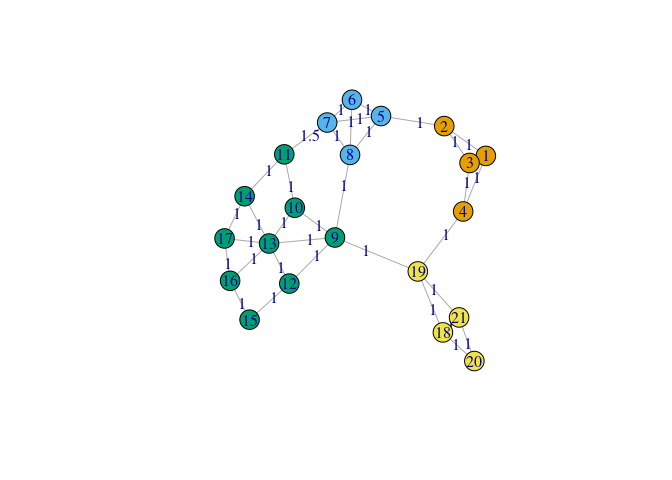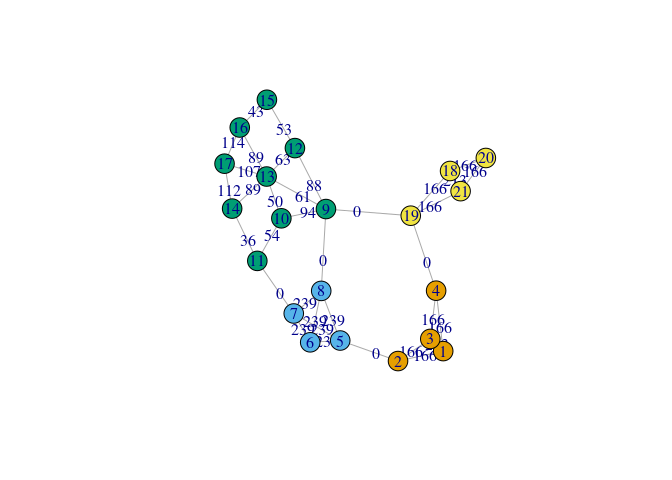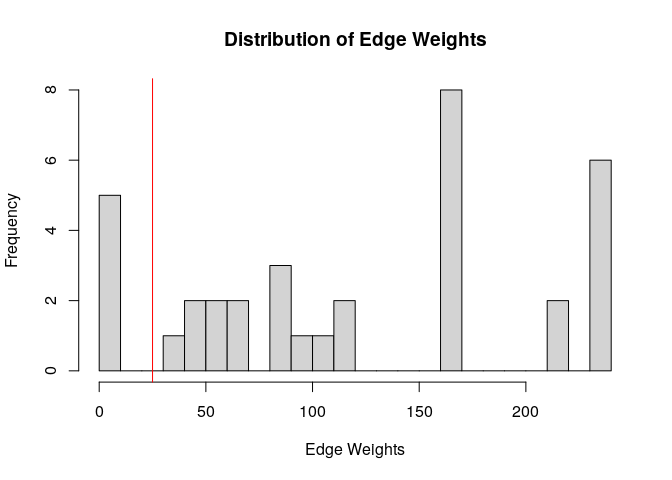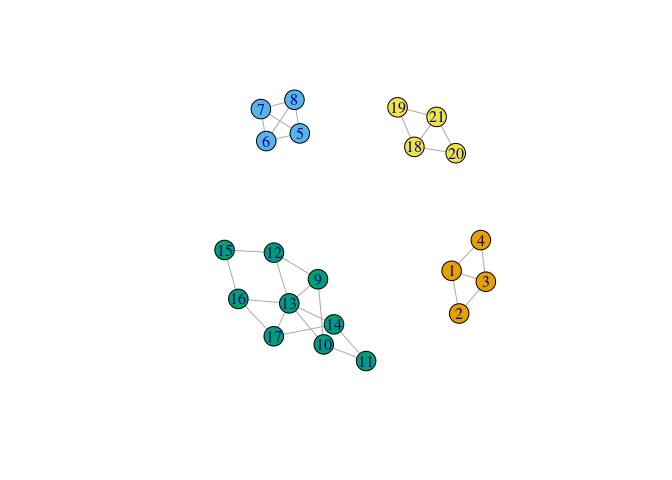# Basic Usage

This vignette shows how to cluster the vertices of a weighted graph using the random walk method developed by Harel and Koren  with the Rwclust package using an example.

library(Rwclust)
library(igraph)

## Introduction

The random walk algorithm is based on the concepts of connected components and cut edges. Let $$G=(V, E)$$ be a graph where $$V$$ is the set of vertices and $$E$$ is the set of edges. A connected component is a subset of vertices that are mutually reachable. A graph can have one connected component constituting the entire graph, or several connected components. If a graph has more than one connected component, the graph is said to be disconnected. In this context, the connected components correspond to clusters.

A set of cut edges is a set $$E' \subset E$$ such that $$G-E$$ is disconnected. Essentially, $$E'$$ contains a set of edges whose removal results in the creation of separate clusters of vertices.

The random walk algorithm finds a set of cut edges but "sharpening" the difference between the weights of edges which connect vertices in within a cluster and the weights of edges that run between clusters. All edges with weights below a certain user-defined threshold are deleted and the resulting connected components become the clusters.

The high-level steps in the algorithm are:

1. sharpen edge weights using random walks
2. select a cutoff using a histogram of the weights
3. delete edges below the cutoff and compute the connected components of the resulting graph

## Usage Example

We will use an example graph taken . The data is contained in a dataframe. We use igraph to create a graph object a display it along with the edge weights.

data(example1, package="Rwclust")
#>   from to weight
#> 1    1  2      1
#> 2    1  3      1
#> 3    1  4      1
#> 4    2  3      1
#> 5    3  4      1
#> 6    2  5      1
labels <- c(1,1,1,1,2,2,2,2,3,3,3,3,3,3,3,3,3,4,4,4,4)

G <- igraph::graph_from_edgelist(as.matrix(example1[, c(1,2)]), directed=FALSE)
G <- igraph::set_edge_attr(G, "weight", value=example1$weight) plot(G, edge.label=E(G)$weight, vertex.color=labels, layout=layout_with_fr)#### 1. Sharpening Edge Weights

The rwclust function applies the edge-sharpening algorithm and returns a list containing a vector of the final weights and a sparse matrix representing the weighted adjacency matrix of the new graph. The iter parameter is the number of algorithm iterations and k is the maximum length of random walk to consider.

result <- rwclust(example1, iter=6, k=3)

Next we plot the new weights. Notice how the weights connecting the clusters are 0 and all the other weights are larger than their original values.

G_sharpened <- igraph::graph_from_edgelist(as.matrix(example1[, c(1,2)]), directed=FALSE)
E(G_sharpened)$weights <- round(weights(result),0) plot(G_sharpened, edge.label=E(G_sharpened)$weights, vertex.color=labels, layout=layout_with_fr)#### 2. Plot the Histogram

Before edges are deleted and the connected components calculated, the user must select a cutoff. To do this we plot a histogram of the edge weights. Note that there appear to be several edges with very small edge weights. 25 seems to be an appropriate cutoff.

plot(result, cutoff=25, breaks=20)#### 3. Delete Edges and Compute Components

The next step is to remove the edges that are below the threshold and compute the connected components.

# delete edges with weights below the threshold
edges_to_keep <- which(weights(result) > 25)
example1_c <- example1[edges_to_keep, ]
example1_c$weight <- weights(result)[edges_to_keep] G_c <- igraph::graph_from_edgelist(as.matrix(example1_c[, c(1,2)]), directed=FALSE) # compute the connected components clusters <- igraph::components(G_c)$membership

plot(G_c, vertex.color=clusters)